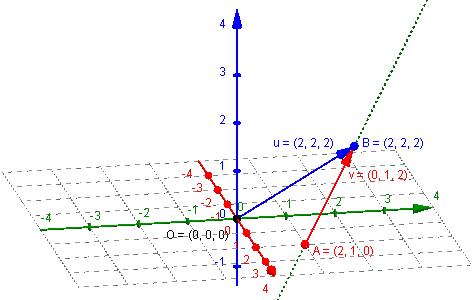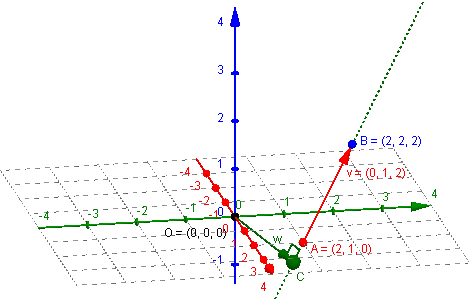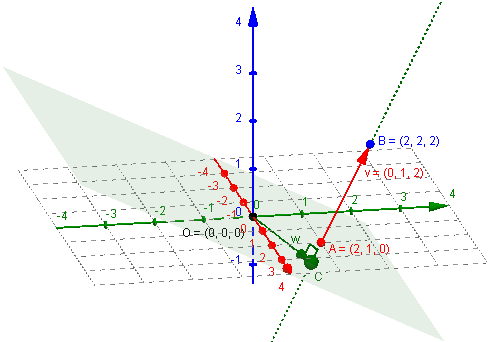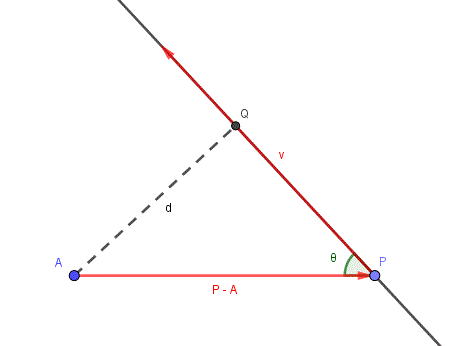# Distance from a Point to a Line in Space

#### (A new question of the week)

Among many interesting recent questions we have one about vectors and equations in three dimensions. We’ll see four different ways to find the distance from a point to a line, proving two formulas and catching some of the errors one can make along the way. We’ll also see a useful collaboration between two of the Math Doctors.

## Distance to a line

This question came from Brian in late March:

I am teaching myself linear algebra using Mary Boas’ book, Mathematical Methods in the Physical Sciences.  I am stumped on the problem of finding the distance between the origin and the line r = 2i + j + (j +2k)t.  I figured that the point (2, 2, 2) is on this line and then tried to take the cross product of the vector from (0, 0, 0) to (2, 2, 2) and the direction vector for this line, which is (0, 1, 2).  But when I take this cross product, it turns out to be zero, which doesn’t make sense since I’m looking for a finite distance.  What am I doing wrong?

Here we can see the line, and Brian’s vectors, u and v:Brian is right that the direction vector for the line is $$\left<0, 1, 2\right>$$, that is, $$0\mathbf{i}+1\mathbf{j}+2\mathbf{k}$$, since that is what is multiplied by the parameter $$t$$. He is also right that the point $$B (2,2,2)$$ is on the line, since that is the point for $$t=1$$, though he could also have used the initial point, $$A (2,1,0)$$ from the position vector $$2\mathbf{i}+\mathbf{j}$$ seen in the equation.

There is a formula using the cross product $$\mathbf{u}\times\mathbf{v}$$ that he refers to, but it doesn’t work quite the way he says. We have to discover where he is thinking incorrectly, and correct what he is doing.

## A second method: perpendicular plane

Doctor Fenton answered by suggesting a different approach using a plane perpendicular to the line:

Hi Brian,

The cross-product shouldn’t be 0, since the two vectors are neither 0 vectors nor parallel, but I don’t think this will be useful for you.  The direction vector of the line will give you a normal vector to any plane perpendicular to the line.  You want a vector from the origin to the line which is perpendicular to the direction of the line.

Can you find the plane perpendicular to the line which passes through the origin?  The intersection of the line with that plane will give you a point on the line which will give you the vector you need.

Here is the vector w representing the distance we want:## A third method: minimizing the distance

Doctor Rick joined in, wanting to correct the method Brian is using, and also suggesting other methods:

Hi, Brian. You certainly need to check your work on the cross product, and if you still get zero, let us see your work so we can identify your error. There are several widely differing ways to find the distance from a point to a line, and one of them does involve the cross product you are doing. It would help if you show the formula or method you are using.

Doctor Fenton suggested a second method. A third method that is particularly appropriate for a parametrically defined line, is to minimize the square of the distance from the point (the origin, here) to the point corresponding to an arbitrary value of t. You may want to stick with the method in the book, but if you want a fuller understanding of the topic, we could discuss all three methods (and maybe even find a fourth).

By the way, I don’t know how you found that the point (2, 2, 2) is on the line, but one way to find such a point is to choose any value for t. Perhaps you picked t = 1; I’d choose t = 0, which gives the point (2, 1, 0), that is, 2i + j. This point is right there in the equation of the line. Any point (any value of t) should give the same result for the distance, however.

We’ll be looking at the third and fourth methods after dealing with Doctor Fenton’s and Brian’s methods.

## Using method 2 (perpendicular plane)

Brian had a response for each Math Doctor. We’ll start with this one, and hold off on what he said to Doctor Rick to avoid juggling two many methods at once.

Thank you, Drs. Fenton and Rick.  Following Dr. Fenton’s method, to find the plane perpendicular to the line and going through the origin, I would substitute the values for a, b, and c, from the direction vector (0, 1, 2) and the point (0, 0, 0) into the equation for a plane.  This gives us 0(x – 0) + 1(y – 0) + 2(z – 0) = 0.  So then the equation of the plane would be y + 2z = 0, which is confusing because we lost the x term.  Also, to find intersection of this plane and the line, don’t I need to have the equation of the plane in parametric form?  I looked up on the internet how to find the parametric equation of a plane; it looks complicated, but if that is the only or best way, I can study it.

The equation of a plane through point $$P (x_1,y_1,z_1)$$ perpendicular to the vector $$\mathbf{v} (a,b,c)$$ is $$a(x-x_1)+b(y-y_1)+c(z-z_1) = 0$$, and Brian has correctly found this equation and simplified it to $$y+2z=0$$. There is nothing wrong with this having no term with x; that just means that it is parallel to the x-axis, so that the x-coordinate is irrelevant.

Here is that perpendicular plane:The goal now is to locate the point I have called C, so we can find the distance OC. We have the equation of the plane, $$y+2z=0$$, and the parametric equation of the line, $$\mathbf{r} = 2\mathbf{i} + \mathbf{j} + (\mathbf{j} +2\mathbf{k})t = 2\mathbf{i} + (1+t)\mathbf{j} + (2t)\mathbf{k}$$. How do we find their intersection? More easily than Brian expects.

Doctor Fenton replied,

No, you don’t need a parametric equation for the plane.  Every point on the line has the form (2, 1+t, 2t), and this point will be in the plane y + 2z = 0 if the sum of the y-coordinate and 2 times the z-coordinate of the point is 0.  That equation in t will give you the value of the parameter t for which the point on the line is in the plane.  The distance from this point to the origin gives you the distance from the point to the line.

Brian found that hint sufficient:

Dr. Fenton, your explanation that every point on the line has the form (2, 1 + t, 2t) and the condition in which this point will be in the plane clarified everything.  So to find the intersection of the line and the plane, start with the equation of the plane y + 2z = 0 and substitute 1 + t for y and 2t for z.  Then solving the equation we get t = -0.2.  Plugging this value of t back into the parametric coordinates of the line, we get (2, 0.8, -0.4) as the point on the line from which we need to calculate the distance to the origin.  The rest is just the distance formula, which gives a distance of 2*sqrt(6/5), which agrees with the answer in the book.  The plane only has two variables in its equation because it coincides with the line x=2.  Thanks much for your invaluable help.

Our point C is $$(2, 0.8, -0.4) = (2,\frac{4}{5}, -\frac{2}{5})$$, and the distance from the origin is $$\sqrt{(2)^2+(\frac{4}{5})^2+(-\frac{2}{5})^2} = \sqrt{4+\frac{16}{25}+\frac{4}{25}} = \sqrt{\frac{120}{25}} = \sqrt{\frac{24}{5}} = 2\sqrt{\frac{6}{5}}$$

So we have solved the problem using Doctor Fenton’s “method 2”. If we rationalize denominators, as is often expected, we get a distance of $$\frac{2\sqrt{30}}{5}$$.

## Using method 1 (cross product formula)

Meanwhile, here is Brian’s earlier response to Doctor Rick, first showing how he got the cross product he said was zero:

As for Dr. Rick’s question, I tried to calculate the cross product of (2, 2, 2) and (0, 1, 2) using the method like calculating a determinant.  I set it up like this

| i   j   k |
| 2  2  2 |
| 0  1  2 |

Then applying the determinant method, I got i(4-2) – j(4-0) + k(2-0), which equals 2i – 4j + 2k.  Then for purposes of finding a distance, I thought I need to take the sum of this expression, which is zero, divided by the norm of the vector, which doesn’t matter if the numerator is zero.  Where am I going wrong?

The cross product is correct: $$\begin{vmatrix}\mathbf{i} &\mathbf{j} &\mathbf{k} \\2 & 2 & 2 \\0 & 1 & 2 \\\end{vmatrix} = (2\cdot 2 – 2\cdot 1)\mathbf{i} – (2\cdot 2 – 2\cdot 0)\mathbf{j} + (2\cdot 1 – 2\cdot 0)\mathbf{k} = 2\mathbf{i} – 4\mathbf{j} + 2\mathbf{k}$$

When Brian said the cross product was zero, we now know what he really meant was that the sum of the components ($$2+(-4)+2=0$$) was zero. But why did he do that?

Doctor Fenton replied, mentioning Doctor Rick’s method 3 and a likely piece of the error:

As Dr. Rick mentioned, you can also use calculus, if you know how to find the minimum of a function, since the distance from the origin to a point on the line is the square root of the sum of the squares of the components of the point, which is the square root of a quadratic polynomial in t.  You can find the minimum of this function using calculus.   (Actually, you can also just minimize the square of the distance, which avoids dealing with the square root, and has a minimum at the same value of t.)

Also, the length of a vector is the square root of the sum of the squares of its components, not just the sum of the components.

This supposes that he added the components as a mistaken way of finding its norm (length). We’ll see that he was right.

Doctor Rick now rejoined the discussion:

Regarding your original method, Brian, I want to be sure you didn’t miss Doctor Fenton’s last remark. You correctly found the cross product (2i + 2j + 2k) × (j + 2k) = 2i – 4j + 2k; you said the cross product “turns out to be zero”, but the cross product is a vector, and it is not the zero vector!

You did not show the formula you were using, but I assume it is something like this: Given a point A and a line defined by a point P on the line and a direction vector v, the (shortest) distance d from A to the line is

d = || (P – A) × v || / || ||

where ||…|| is the length (norm or magnitude) of a vector. As Doctor Fenton pointed out, the length is the square root of the sum of the squares of the vector components, not the sum of the components.

The numerator of this formula is the cross product Brian found (between P – A, which I called u, and direction vector v); so, yes, he added its components as an attempt to find its norm.

Brian responded, correcting his work:

Thank you for following up about this, Dr. Rick.  Yes, the formula you indicated is the one I was using, and yes, I had not adequately absorbed what Dr. Fenton said.  I went back and recalculated the distance in light of all this feedback and now the answer came out right.

That said, I also want to say that the way Dr. Fenton explained the problem and how to solve it makes sense to me intuitively and is something I can visualize geometrically.  While I got the correct answer using the method shown in my textbook, with Dr. Fenton’s and your help, I still can’t visualize why the norm of the cross product (P-A) and v divided by the norm of v should give us the distance we are looking for.  I’m not asking you to necessarily explain this (though if you have an explanation I’m all ears), only noting that I found Dr. Fenton’s approach more intuitively understandable.

Here is the working of the formula: $$\frac{\left\| \mathbf{u}\times\mathbf{v} \right\|}{\left\| \mathbf{v} \right\|}=\frac{\left\| 2\mathbf{i} – 4\mathbf{j} + 2\mathbf{k} \right\|}{\left\| 0\mathbf{i} + 1\mathbf{j} + 2\mathbf{k} \right\|}=\frac{\sqrt{2^2+(-4)^2+2^2}}{\sqrt{0^2+1^2+2^2}}=\frac{\sqrt{24}}{\sqrt{5}} = \frac{2\sqrt{30}}{5}$$

### Proving the formula algebraically

But he wants an explanation to make the formula more understandable, and therefore more memorable.

Doctor Fenton replied with an algebraic explanation:

Using the notation from earlier, the point A is the given point not on the line, P an arbitrary point on the line, and v the vector direction of the line.

Suppose Q is the point on the line closest to A.  The vector w = QA is perpendicular to the line, so its length ||w|| is the distance from A to the line.

Then the vector u = P – A is the sum of the vector w and some multiple of v: u = w + kv .

The cross-product u × v = (w + kv) × v = w × v + k v × v = w × v because the cross-product v × v = 0.

The length of ||u × v|| = ||w × v|| = ||w|| ||v|| sin θ, where θ is the angle between v and w.

But w and v are perpendicular, so θ = π/2 and sin θ = 1.  Therefore the distance d from A to the line is

d = ||w|| = ||u × v|| / ||v|| = ||(P – A) × v|| / ||v||.

The key idea here is that the cross product of u and v is the same as the cross product of w and v, so that without actually finding Q, we can find its distance from A. (This w is not the same as my w = OC in an earlier picture.)

Thank you, Dr. Fenton.  This is very helpful and gives me a solid algebraic understanding of the formula.  But I am still trying unsuccessfully to visualize what all this looks like geometrically.  I am envisioning a line with P and Q on it and a point A not on the line but closest to the line at point Q.  All of this fits into a two dimensional picture.  But in that case, wouldn’t the cross product of vector AP and v be a vector coming out of the page?  But we are looking for the distance from Q to A, which is a vector in the plane of the page.  That is what I’m confused about, but maybe I am just having trouble thinking about the problem in three dimensions.

He is right on all counts! Doctor Fenton’s proof gives no direct geometrical understanding. But …

### Understanding the formula geometrically

Doctor Rick now helped with that:

Hi, Brian. You’re right that the cross-product vector is perpendicular to the plane containing A and the line. However, the formula uses only the magnitude of the cross-product. The distance calculated by the formula is not a vector but a length (the magnitude of AQ).

I am attaching a figure; the formula uses the fact that the magnitude of the cross-product is ||P-A|| ||v|| sin θ, and the distance d is ||P-A|| sin θ from right-triangle trigonometry.Our vector w is a vector perpendicular to this plane, but with the same length as d. Why? Because of how the cross product works.

Taking $$\mathbf{u} = P-A$$, the distance $$d=\left\|\mathbf{u}\right\|\sin\theta$$.

But the magnitude of $$\mathbf{w}=\mathbf{u}\times \mathbf{v}$$ is $$\left\|\mathbf{u}\right\| \left\|\mathbf{v}\right\|\sin\theta$$, so (from the right triangle APQ) $$d=\left\|\mathbf{u}\right\|\sin\theta = \frac{\left\|\mathbf{u}\right\| \left\|\mathbf{v}\right\|\sin\theta}{\left\|\mathbf{v}\right\|}= \frac{\mathbf{u}\times \mathbf{v}}{\left\|\mathbf{v}\right\|}$$. And that’s the formula.

Brian closed the discussion:

Thank you, Dr. Rick.  Your diagram is helpful and your distinction between the norm of the cross product and the vector itself is very clarifying.  I think I am in business with all this now.  Dr. Fenton’s explanation of the formula is helpful for problems in which we don’t know or want to know the acute angle QPA and your explanation is helpful for problems in which the acute angle is in play.

Both explanations actually used the angle; and we don’t need to think of it to apply the formula. But it is good to have both a primarily algebraic proof and a visual understanding of it.

But there is more to look at! We left a couple things dangling.

## A fourth method: projection on the line

First, the last picture suggests another approach we could take to find the distance d: Rather than use the cross product and the sine of the angle, we could use the dot product and the cosine of the angle. This will allow us to find point Q, which the cross product formula doesn’t do; but the formula will be considerably more complicated.

We can find point Q as the orthogonal projection of A on the line. To do this, notice that $$PQ = \left\|\mathbf{u}\right\|\cos\theta$$ But $$(-\mathbf{u})\cdot \mathbf{v} = \left\|\mathbf{u}\right\| \left\|\mathbf{v}\right\|\cos\theta$$ Therefore, $$PQ = -\frac{\mathbf{u}\cdot \mathbf{v}}{\left\|\mathbf{v}\right\|}$$

So we can find Q as P plus a vector with this length in the direction of v. So we just have to multiply the unit vector in the direction of v by the magnitude we just found: $$Q = P-\frac{\mathbf{u}\cdot \mathbf{v}}{\left\|\mathbf{v}\right\|}\frac{\mathbf{v}}{\left\|\mathbf{v}\right\|} = P-\frac{\mathbf{u}\cdot \mathbf{v}}{\left\|\mathbf{v}\right\|^2}\mathbf{v}$$

Then our distance d is the magnitude of vector w = AQ, which is $$Q-A = (P-A)-\frac{\mathbf{u}\cdot \mathbf{v}}{\left\|\mathbf{v}\right\|^2}\mathbf{v} = \mathbf{u}-\frac{\mathbf{u}\cdot \mathbf{v}}{\left\|\mathbf{v}\right\|^2}\mathbf{v}$$

For our specific problem, we find that $$\mathbf{w}=\mathbf{u}-\frac{\mathbf{u}\cdot \mathbf{v}}{\left\|\mathbf{v}\right\|^2}\mathbf{v}=\left<2, 1, 0\right>-\frac{\left<2, 1, 0\right>\cdot \left<0, 1, 2\right>}{\left\|\left<0, 1, 2\right>\right\|^2}\left<0, 1, 2\right> = \left<2, 1, 0\right>-\frac{1}{0^2+1^2+2^2}\left<0, 1, 2\right>\\ = \left<2, 1, 0\right>-\frac{1}{5}\left<0, 1, 2\right> = \left<2, 1, 0\right>-\left<0, \frac{1}{5}, \frac{2}{5}\right> = \left<2, \frac{4}{5}, -\frac{2}{5}\right>$$ This is the same point we’ve seen before, so we get the correct distance.

## Using method 3 (minimizing distance)

We never got back to the calculus approach; likely Brian is not in a position to use it. But since it was mentioned, let’s carry it out.

We have the equation of the plane, $$y+2z=0$$, and the parametric equation of the line, $$(x,y,z) = (2, 1+t, 2t)$$, and we want to find the distance to the line from the origin. For a given t, the distance from the origin is $$\sqrt{2^2+(1+t)^2+(2t)^2} = \sqrt{4+(1+2t+t^2)+4t^2} = \sqrt{5t^2+2t+5}$$ The square root will be minimized when the radicand is minimized, so we just need to minimize $$f(t) = 5t^2+2t+5$$.

Taking the derivative, $$f'(t) = 10t + 2$$, which is zero for $$t=-\frac{1}{5}$$. This yields the point $$(x,y,z) = \left(2, 1-\frac{1}{5}, -2\frac{1}{5}\right) = \left(2, \frac{4}{5},-\frac{2}{5}\right)$$. That’s the same point we’ve seen twice now. And its distance from the origin, as we saw before, is $$\frac{2\sqrt{30}}{5}$$.

This turned to be the easiest of all; but since Brian is studying vector methods, let’s not tell him that.

### 3 thoughts on “Distance from a Point to a Line in Space”

1.Rick Peterson (Doctor Rick)

I’d like to add that Method 3 does not actually require calculus. The function $$y = 5t^2 + 2t + 5$$ (where y is the square of the distance) is the equation of a parabola; the minimum (squared) distance is the ordinate of the vertex of this parabola. Algebra students learn to find the vertex of a parabola by completing the square: $$y = 5(t + 1/5)^2 – 1/5 + 5$$, so the minimum occurs at $$t = -1/5, y = 24/5$$. Thus the minimum distance is $$\sqrt{24/5} = 2\sqrt{6/5} = 2\sqrt{30}/5$$ (once again).

So Brian could have done this, I’m sure, but two methods were enough for him.

1.I think you have a typo in your comment here: the minimum is at t = -1/5 (you missed the negative sign).

–Dr. Schwa

PS: If the Ask Dr. Math archives are available anywhere but the wayback machine at this point, I’d love to know! The links on the homepage here are to defunct NCTM pages.

1.Thanks for the correction; I’ve taken care of it.

It’s nice to hear from you. Discussions are under way for setting Ask Dr. Math free and making it accessible again, but it takes time. For the summer, I’ll be posting only new answers, and hoping things will return to normal after that.

This site uses Akismet to reduce spam. Learn how your comment data is processed.